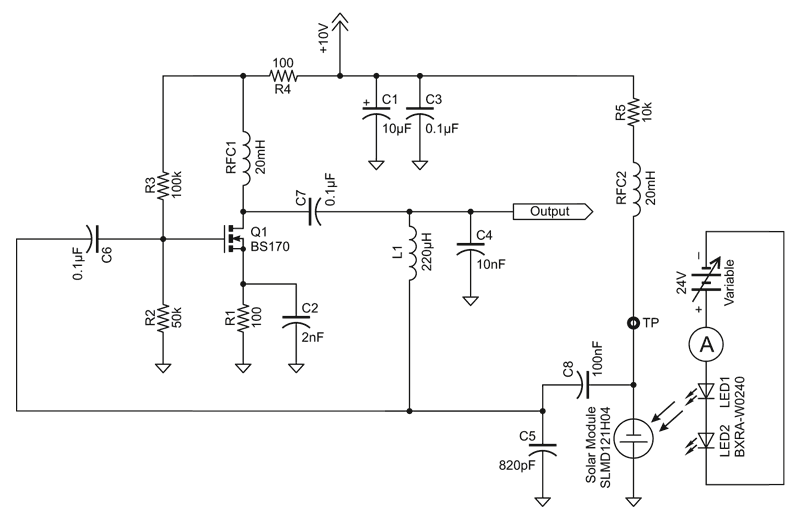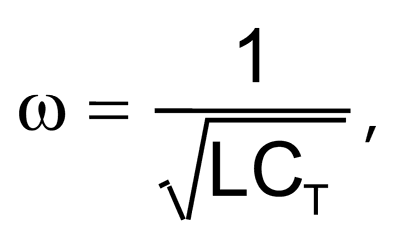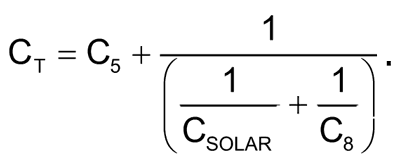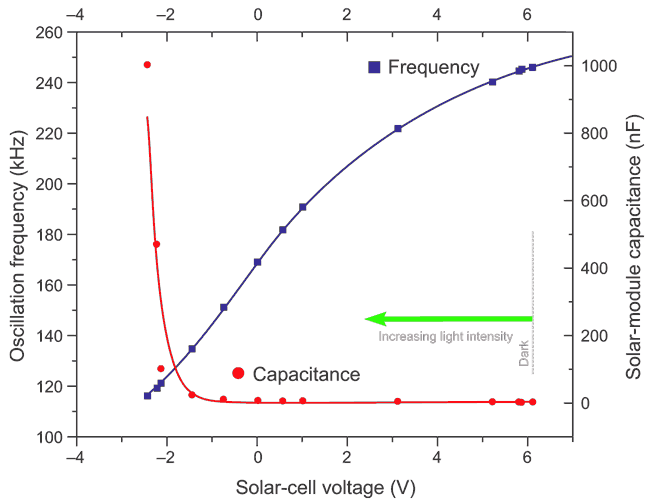# Light-controlled oscillator uses solar cell

EDN

Any PN junction diode can be used as a variable capacitor in reverse biased mode, as well as in the forward biased mode before the knee voltage. The capacitance is usually very small as the junction size is small. Though the varactor diode is specially designed for higher capacitance, it's still limited to a few hundred picofarads. A solar cell can also be used in some circuits as a variable capacitor. As its junction size is much larger than the usual diodes, much larger variation of capacitance is expected. There is a drawback using a solar cell as a capacitor: the reverse saturation current is also much higher, causin it to act like a leaky capacitor. Still, a solar cell can be used in some oscillator ciruits to achieve a widely tunable frequency.Figure 1. Light-controlled Pierce oscillator.

A Pierce oscillator was implemented using a BS170 MOSFET and a positive feedback network consisting of L1, C4, and C5 (Figure 1). The amplifier gain was adjusted, choosing bypass capacitor C2 = 2 nF, for nearly sinusoidal output at an oscillation frequency around 250 kHz. A solar module (IXYS SLMD121H04, open circuit voltage: 2.52 V; short circuit current: 50 mA) was connected in series with C8 (100 nF) to capacitor C5. When the solar-module capacitance (CSOLAR) varies, the series equivalent of CSOLAR and C8 paralleled with C5, also changes. The frequency of oscillation is given by the following equation:whereTwo power LEDs (Bridgelux: BXRA-W0240) connected in series were used to illuminate the solar module, powered by a 24 V variable power supply. The LEDs were mounted on a heatsink and were placed ~ 5 cm above the solar module. The whole area of the solar module (43 mm × 14 mm) was illuminated uniformly by the LEDs. Voltage across the solar module terminals was also measured at testpoint TP with changing illumination.

Initially, the solar module was put inside a small black box, and the voltage at TP read 6.2 V, giving dark saturation current of 380 µA. Frequency of oscillation under darkness was 246 kHz. With only ambient light, the frequency reduced to 245 kHz. As the LED current was increased, oscillation frequency continued going down; at 330 mA, the frequency was 116 kHz. Beyond a LED current of 330 mA, the total capacitance, CT, was not changing much, leaving the tuning range from 246 to 116kHz. The tuning characteristic is shown in Figure 2.Figure 2. Solar cell voltage vs. capacitance & frequency.

The solar module capacitance was approximated by putting different capacitances in place of the solar module. In a separate test, short circuit current for the solar module was measured under illumination, keeping the same separation (~ 5 cm). At 330 mA LED current, the corresponding short circuit current of the solar module was 14.5 mA; the light intensity was found from the data sheet of the solar module to be ~ 400 W/cm2.

It should be mentioned that throughout the tuning range, the output amplitude varied as the closed loop gain varied due to changing capacitance and leakage in solar module. To achieve constant amplitude, an additional circuit would be needed.

References

1. G. Gonzalez, “Foundations of Oscillator Circuit Design”, Artech House Inc., MA, USA, 2007.

Appendix 1. Solar cell capacitance

 Voltage (V) Frequency (kHz) Capacitance (nF) 6.1 246.1 1.45 5.86 245.4 1.5 5.8 244.7 1.6 5.21 240.2 1.8 3.11 221.8 3 1 190.7 4 0.55 181.6 4.7 0 169 6 -0.76 151.1 10 -1.46 134.5 22 -2.15 121 100 -2.24 119 470 -2.45 116 1000

EDN